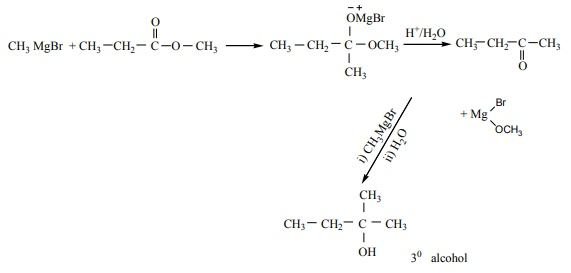# Chemistry - Online Test

Q1. Which of the following is an analgesic?
Explaination / Solution:
No Explaination.

Q2. Concentration of the Ag+ ions in a saturated solution of Ag2 C2O4 is 2.24 ×10-4mol L-1 solubility product of Ag2 C2O4 is
Explaination / Solution:

Ag2C2O4 2Ag+ + C2O42-

[ Ag+ ] = 2.24 × 10-4 mol L-1

[ C2 O42- ] = { 2.24×10-4 }/2 = mol L-1

= 1.12×10-4 mol L-1

Ksp = [Ag+]2[C2O42-]

= (2.24×10-4 mol-4 L-1 )2 (1.12×10-4 mol L-1)

= 5.619×10-12mol3 L-3

Q3. The number of electrons that have a total charge of 9650 coulombs is
Explaination / Solution:

1F = 96500 C = 1 mole of e = 6.023 ×1023 e

9650 C = [ 6.22 ×1023 / 96500 ] × 9650 = 6.022×1022

= 6.022×1022

Q4. Which of the following is incorrect for physisorption?
Explaination / Solution:

The incorrect statement is option (b)

Physisorption is an exothermic process. Hence increase in temperature decreases the physisorption.

Q5. Which of the following compounds on reaction with methyl magnesium bromide will give tertiary alcohol.
Explaination / Solution:Q6. The segments of leech are known as
Explaination / Solution:
No Explaination.

Q7. Water which is absorbed by roots is transported to aerial parts of the plant through
Explaination / Solution:
No Explaination.

Q8. The formation of cyanohydrin from acetone is an example of
Explaination / Solution:
No Explaination.

Q9. Site for processing of vision, hearing, memory, speech, intelligence and thought is
Explaination / Solution:
No Explaination.

Q10. The hormone which has positive effect on apical dominance is:
Explaination / Solution:
No Explaination.